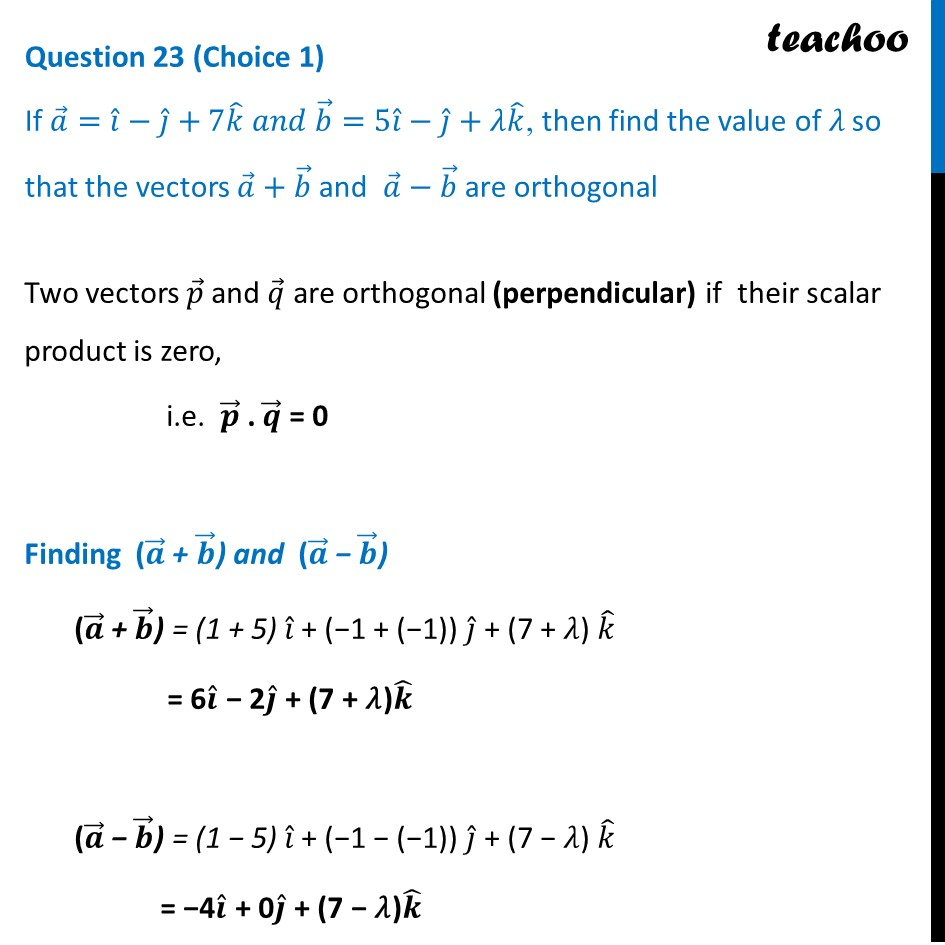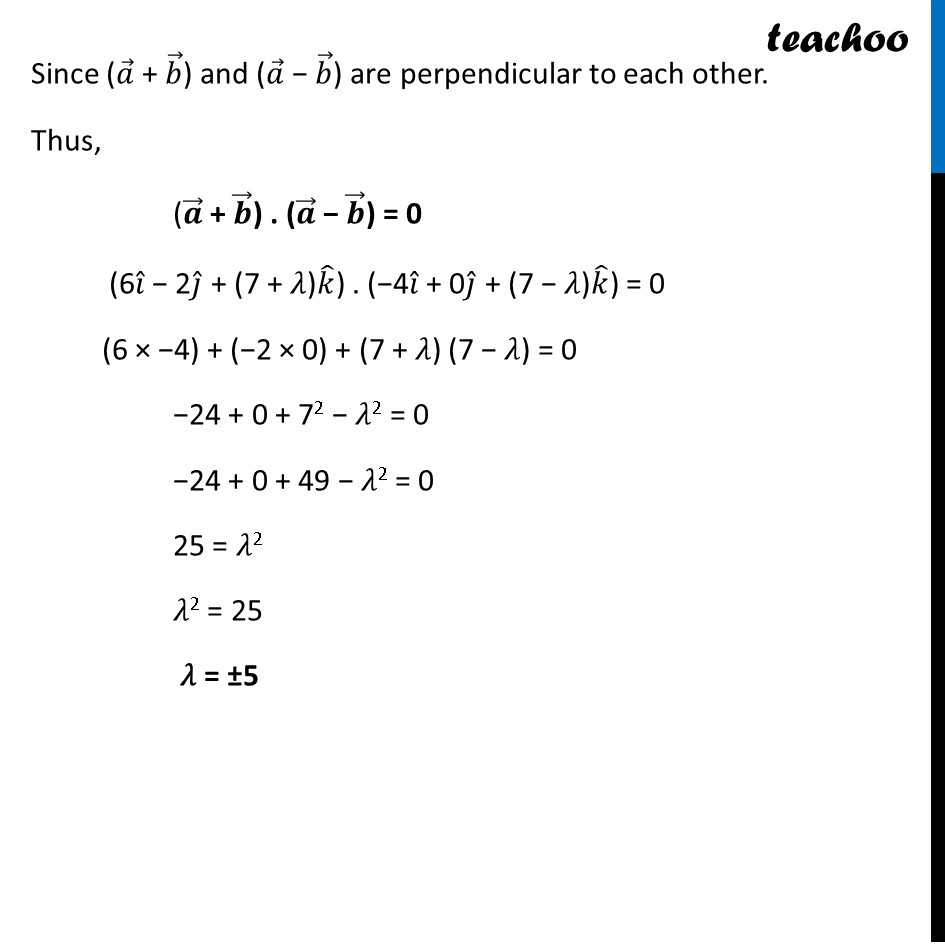CBSE Class 12 Sample Paper for 2023 Boards

Class 12
Solutions of Sample Papers and Past Year Papers - for Class 12 Boards

## If a = i ̂ - j ̂ + 7k ̂ 𝑎𝑛𝑑 b ⃗= 5i ̂ - j ̂ + λk ̂, then find the value of 𝜆 so that the vectors a ⃗ + b ⃗ and  a ⃗ - b ⃗ are orthogonalLearn in your speed, with individual attention - Teachoo Maths 1-on-1 Class

### Transcript

Question 23 (Choice 1) If 𝑎 ⃗=𝑖 ̂−𝑗 ̂+7𝑘 ̂ 𝑎𝑛𝑑 𝑏 ⃗=5𝑖 ̂−𝑗 ̂+"𝜆" 𝑘 ̂, then find the value of 𝜆 so that the vectors 𝑎 ⃗+𝑏 ⃗ and 𝑎 ⃗−𝑏 ⃗ are orthogonal Two vectors 𝑝 ⃗ and 𝑞 ⃗ are orthogonal (perpendicular) if their scalar product is zero, i.e. 𝒑 ⃗ . 𝒒 ⃗ = 0 Finding (𝒂 ⃗ + 𝒃 ⃗) and (𝒂 ⃗ − 𝒃 ⃗) (𝒂 ⃗ + 𝒃 ⃗) = (1 + 5) 𝑖 ̂ + (−1 + (−1)) 𝑗 ̂ + (7 + 𝜆) 𝑘 ̂ = 6𝒊 ̂ − 2𝒋 ̂ + (7 + 𝜆)𝒌 ̂ (𝒂 ⃗ − 𝒃 ⃗) = (1 − 5) 𝑖 ̂ + (−1 − (−1)) 𝑗 ̂ + (7 − 𝜆) 𝑘 ̂ = −4𝒊 ̂ + 0𝒋 ̂ + (7 − 𝜆)𝒌 ̂ Since (𝑎 ⃗ + 𝑏 ⃗) and (𝑎 ⃗ − 𝑏 ⃗) are perpendicular to each other. Thus, (𝒂 ⃗ + 𝒃 ⃗) . (𝒂 ⃗ − 𝒃 ⃗) = 0 (6𝑖 ̂ − 2𝑗 ̂ + (7 + 𝜆)𝑘 ̂) . (−4𝑖 ̂ + 0𝑗 ̂ + (7 − 𝜆)𝑘 ̂) = 0 (6 × −4) + (−2 × 0) + (7 + 𝜆) (7 − 𝜆) = 0 −24 + 0 + 72 − 𝜆2 = 0 −24 + 0 + 49 − 𝜆2 = 0 25 = 𝜆2 𝜆2 = 25 𝜆 = ±5